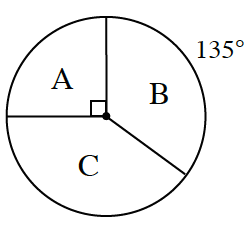### Home > CCG > Chapter 5 > Lesson 5.1.2 > Problem5-20

5-20.

The spinner at right has three regions: A, B, and C. To play the game, you must spin it twice. If the game were played $80$ times, how many times would you expect to get A on both spins? Use a tree diagram or area model to help you answer the question.

What is $P(\text{A})$ for one spin?
Remember how many degrees are in a circle.

Are the spins independent of each other? That means the first spin has no effect on the outcome of the second spin.

What is the probability of spinning an A on the first spin? Multiply by the probability of spinning an A on the second spin.

Multiply the $P(\text{A and A})$, by $80$ to find how many times you would expect to get A on both spins during the $80$ trials.

$5$ times